Notebook

# Market Panic: Parameter-Optimized Strategy for Lay Investors¶

Spurred by a recent 538 piece on whether to sell or to hold during a market panic, i.e. when the S&P suffers a big decline, I wrote an algorithm that allows users to test simple strategies trading SPY depending on price movements. These strategies fundamentally depend on two parameters:

1. n, the percent decrease from a recent high (as a sell-signal)
2. m, the percent increase from a recent low, or an equivalent metric (as a buy-signal)

In this notebook, I'll try to find the optimal (m,n) pair, and comment on the worthwhileness of such a strategy.

In :
# import necessary libraries
from matplotlib import pyplot
from zipline import TradingAlgorithm
from zipline.api import order, record, symbol, history, add_history
import seaborn as sns
import numpy as np
import pandas

In :
# retrieve pricing data for our algorithm
data = get_pricing(['SPY'],
start_date='2002-01-01',
end_date = '2015-02-15',
frequency='daily')
data = data.transpose(2,1,0)


First, we need a benchmark. Let's see how much simply holding SPY returned over the time period (2002-2015).

In :
# algorithm to buy as much SPY as possible on the first day, and then to hold
def initialize(context):
context.day=0

def handle_data(context,data):
if context.day == 0:
price = data[symbols('SPY')].close_price
target_number = context.portfolio.cash / price
context.first_target = target_number
order(symbols('SPY'),target_number)
context.day +=1

In :
# run the algorithm.
handle_data=handle_data)
perf_manual = algo_obj.run(data)
spy_returns = perf_manual.returns.sum()
print spy_returns

0.8534137144


So SPY returned about 0.85 between January 2002 and February 2015, i.e. went up by 85%. Note that it is not standard to look at returns as the measure for an algorithm's success (instead, we prefer to look at Sharpe and Beta). In this case, it is defensible because we're trading SPY (which is the benchmark that Sharpe and Beta are usually measured against). See the Quantopian Lecture Series for other metrics.

Now we create a trading algorithm that buys SPY in January 2002, sells when it drops by n%, and buys back when it rebounds by m%. (Feel free to play with this algorithm -- it could use other simple signals to buy and sell.) It is worth noting that this is implicitly a momentum algorithm: when we have a drop of n%, we bet that SPY will decline further, and when we have a rebound of m%, we bet that SPY will rise further.

In [ ]:
# The trading algorithm itself
def initialize(context):
context.n = n
context.m = m
context.day = 0
context.max_price = 0
context.min_price = 1000000
context.spy = symbol('SPY')
context.holding = False
context.first_target = 0

def handle_data(context, data):
price = data[context.spy].close_price

# on the first day, buy as many shares as possible
if context.day == 0:
context.max_price = price
target_number = context.portfolio.cash / price
context.first_target = target_number
order(context.spy,target_number)
context.holding = True
context.day +=1

if context.holding:
if price > context.max_price:
context.max_price = price
elif price < context.max_price * (1 - context.n):
context.min_price = price
held = context.portfolio.positions[context.spy].amount
order(context.spy,-held)
context.holding = False
else:
# uncomment next line if testing the alternate model
# context.portfolio.cash *=  1.004369
if price < context.min_price:
context.min_price = price
if price > context.min_price * (1 + context.m):
# an alternate condition to catch upward recovery trends and turn a profit
#if price > context.min_price and price >= context.max_price * (1 - n) * (1 - m):
context.max_price = price
target_number = context.portfolio.cash / price
order(context.spy,target_number)
context.holding = True


Now we run the algorithm for n and m up to 25% -- beyond that, occurrences become too rare to draw any conclusions.

Beware of running the code below -- it's 2500 backtests, which will take many hours to complete.

In [ ]:
# list of n- and m-values
ms = [0.000, 0.005, 0.010, 0.015, 0.020, 0.025, 0.030, 0.035, 0.040, 0.045, 0.050, 0.055, 0.060, 0.065,
0.070, 0.075, 0.080, 0.085, 0.090, 0.095, 0.100, 0.105, 0.110, 0.115, 0.120, 0.125, 0.130, 0.135,
0.140, 0.145, 0.150, 0.155, 0.160, 0.165, 0.170, 0.175, 0.180, 0.185, 0.190, 0.195, 0.200, 0.205,
0.210, 0.215, 0.220, 0.225, 0.230, 0.235, 0.240, 0.245, 0.250]

ns = [0.000, 0.005, 0.010, 0.015, 0.020, 0.025, 0.030, 0.035, 0.040, 0.045, 0.050, 0.055, 0.060, 0.065,
0.070, 0.075, 0.080, 0.085, 0.090, 0.095, 0.100, 0.105, 0.110, 0.115, 0.120, 0.125, 0.130, 0.135,
0.140, 0.145, 0.150, 0.155, 0.160, 0.165, 0.170, 0.175, 0.180, 0.185, 0.190, 0.195, 0.200, 0.205,
0.210, 0.215, 0.220, 0.225, 0.230, 0.235, 0.240, 0.245, 0.250]

backtest_count = 0
returns = pandas.DataFrame(index=ns,columns=ms)

for n in ns:
for m in ms:
handle_data=handle_data)
perf_manual = algo_obj.run(data)
backtest_count += 1
print("Backtest {0} completed...").format(backtest_count)
# grab the most recent return. careful to assign column-first
returns[m][n] = perf_manual.returns.sum()


I saved the results obtained from the above process in a local spreadsheet, which I'll load in next. The spreadsheet is a table of returns for every pair of (m,n) values.

In :
# to retrieve and format spreadsheet
def get_csv(filename):
r = local_csv(filename)
r = r.iloc[:,1:]
r.index = list(r.columns)
return r

In :
returns_nm = get_csv("returns_nm.csv")


There's a good tool we can use to visualize this data: a heatmap.

In :
# to create our heatmap
def heat_map(df,cmap='RdYlGn'):
ax = sns.heatmap(df,cmap=cmap)
ax.set_xlabel("m (% rebound)")
ax.set_ylabel("n (% drop)")

In :
heat_map(returns_nm)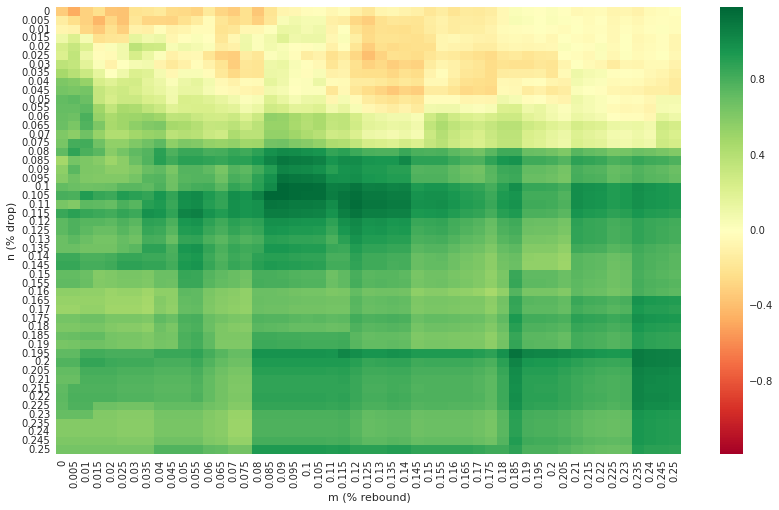We can see that trading on a drop of less than 7% (n $\leq$ 0.07) is generally a bad idea -- the reason for this is probably that SPY's movements are to some extent noisy, so trading on small movements is to trade on noise, which can be expected to go poorly. Aside from that, it looks as if decent returns are achieved by some (m,n) pairs.

What we're interested in are the good returns. Let's filter the above heatmap only down to the returns that are greater than or equal to the SPY returns we found earlier. We'll use a slightly different colormap to avoid confusion.

In :
returns_nm_filtered = returns_nm[returns_nm >= spy_returns]
heat_map(returns_nm_filtered,cmap='summer')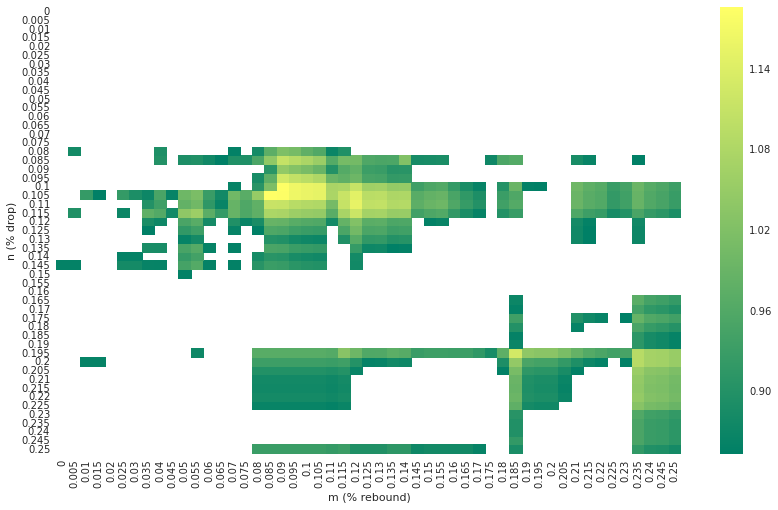We can definitely see that a large number of (m,n) values have returns that outperform SPY. In particular, a maximum appears to be achieved around m=0.105, n=0.085. Let's get that figure:

In :
returns_nm_max = returns_nm.max().max()
print returns_nm_max

1.186085


So: should we run this trading algorithm with these (m,n) values? We should note that between January 2002 and February 2015, there have really only been two major economic downturns. As such, our strategy is working on a limited sample size, so we can expect that these (m,n) values are somewhat overfit -- we would have to expect that this strategy might work, but not quite as well on the next economic downturn.

A further consideration is that in our trading strategy, we only bought and sold SPY, which means that we were occasionally holding a large lump of cash. Realistically, we should invest that cash in something else while we're divested from SPY. I reasoned that such investments could probably return 3% a year -- the risk-free rate of returns is 2%, and even in a time of economic crisis, it's likely that a lay investor can make another 1% by some means.

Dividing 3% by 252 trading days creates a daily return of about 0.004369. I adjusted the trading algorithm to incorporate this return on every day that the portfolio is not holding SPY. This resulted in the following heatmap of returns:

In :
returns_nm_a = get_csv("returns_nm_alternate.csv")
heat_map(returns_nm_a)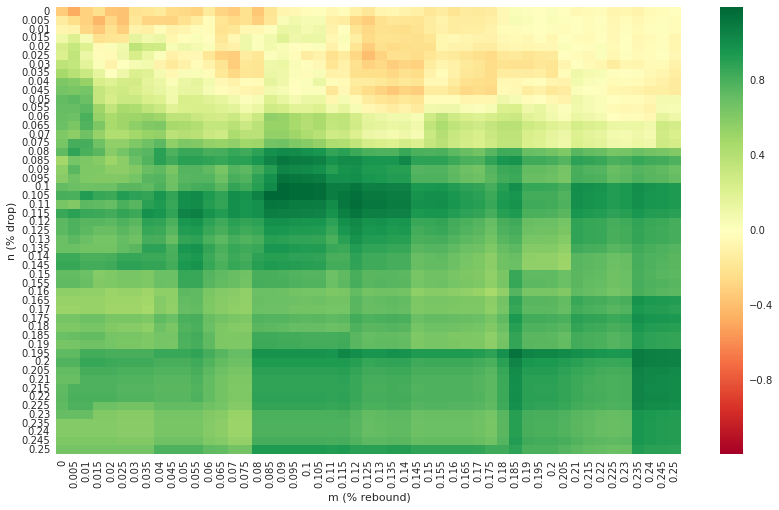This looks very similar to the heatmap for the regular algorithm. Let's filter and find the max:

In :
returns_nm_a_filtered = returns_nm_a[returns_nm_a >= spy_returns]
heat_map(returns_nm_a_filtered,cmap='summer')
returns_nm_a_max = returns_nm_a.max().max()
print returns_nm_a_max

1.190777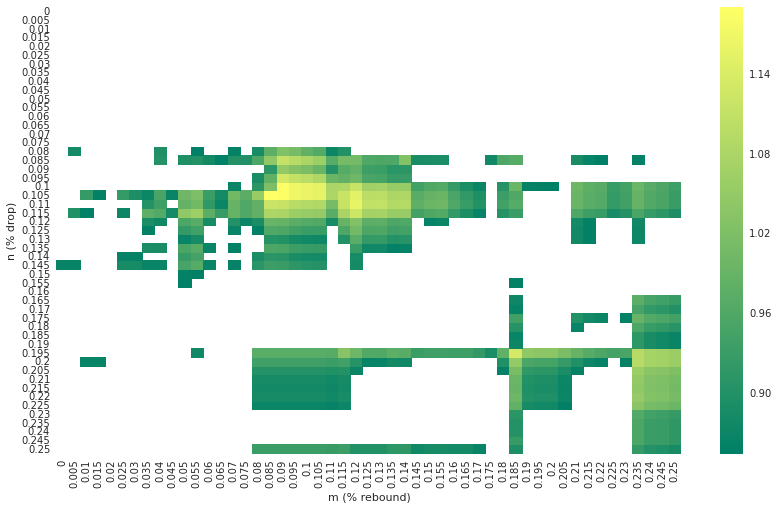Here we see a return of 1.191 (rounded) -- corresponding to a 119.1% gain. For the regular algorithm, we had a gain of 118.6%. Comparing the heatmaps, we can see that the augmentation we made to the algorithm in letting investors gain while not invested in SPY makes a negligible difference.

I considered augmenting the algorithm to let the investor short SPY upon a particular signal, but decided against it for two reasons:

1. I'm trying to answer a question for lay investors who don't have the resources to engage in a more sophisticated strategy.
2. As a long-term strategy, the occasional shorting of the market is quite risky, because the market trends up over time.

Nonetheless, the (m=0.105, n=0.085) strategy seems compelling at a glance. Over thirteen years, it returned 119%, as opposed to SPY's 85%. Let's see how it performed, exactly:

In :
# The trading algorithm itself
def initialize(context):
context.n = 0.105
context.m = 0.085
context.day = 0
context.max_price = 0
context.min_price = 1000000
context.spy = symbol('SPY')
context.holding = False
context.first_target = 0

def handle_data(context, data):
price = data[context.spy].close_price

# on the first day, buy as many shares as possible
if context.day == 0:
context.max_price = price
target_number = context.portfolio.cash / price
context.first_target = target_number
order(context.spy,target_number)
context.holding = True
context.day +=1

if context.holding:
if price > context.max_price:
context.max_price = price
elif price < context.max_price * (1 - context.n):
context.min_price = price
held = context.portfolio.positions[context.spy].amount
order(context.spy,-held)
context.holding = False
else:
context.portfolio.cash *=  1.004369
if price < context.min_price:
context.min_price = price
if price > context.min_price * (1 + context.m):
context.max_price = price
target_number = context.portfolio.cash / price
order(context.spy,target_number)
context.holding = True
record(SPY = context.first_target * data[context.spy].close_price)

In :
def analyze(context, perf):
fig = pyplot.figure()
perf.portfolio_value.plot(ax=ax1, figsize=(16,12))
ax1.set_ylabel('Portfolio Value in $') perf['SPY'].plot(ax=ax1, figsize=(16, 12),c='#B21305') pyplot.legend(loc=0,labels=['M/N Strategy','Hold SPY']) pyplot.show()  In : # Create algorithm object passing in initialize and # handle_data functions algo_obj = TradingAlgorithm( initialize=initialize, handle_data=handle_data ) # HACK: Analyze isn't supported by the parameter-based API, so # tack it directly onto the object. algo_obj._analyze = analyze # Run algorithm perf_manual = algo_obj.run(data)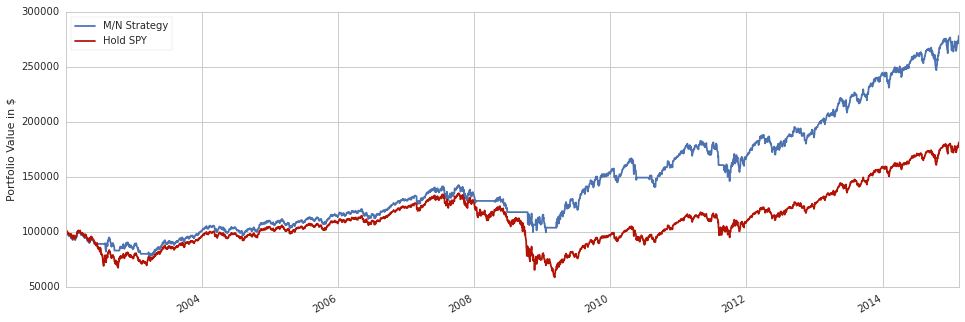Now we can see why this algorithm so strongly outperforms SPY: it avoided the great recession. Before 2008, it very slightly outperformed SPY. The big difference is that the M/N Strategy did not lose nearly as much value at the start of the great recession because it sold after losing 8.5%. Thus, at the very bottom of the recession, the M/N strategy portfolio was worth a little over \$100k, whereas the buy-and-hold SPY portfolio was worth about \\$60k. At that point, both strategies invested fully in SPY and rode the bull market over the last five years. Throughout those five years, the market gained about 150%, so it makes sense that the M/N strategy had much higher total returns.

Does that mean that the M/N Strategy is necessarily better? No: outperforming because you happen to have more cash at the start of a phenomenal bull market is not particularly significant. The M/N Strategy performed so strongly because of only one reason: it managed to save a lot of money during the recession (which in turn left it with more cash on hand at the start of the recovery).

Now that we've established that the success of this strategy was basically dependent on one event, we immediately start to think of an overfit. And, looking at the heatmap, it is eerie that this strategy should be so successful with m=0.085 and n=0.105, but with m=0.075 and n=0.100, it would underperform SPY. Those percentage differences are tiny: one percent and half a percent, respectively! That's well within variation that could be caused by noise.

What will the next major economic downturn be like? I don't know, but I expect that it will be structurally quite different from the one we saw in 2008. As such, we cannot apply the strategy we discussed above, because we learned two things:

• The best M/N strategy was effectively overfit to one event. That's statistically extremely unsound to use for future prediction.
• A small amount of noise could have completely thrown off the strategy and made it comparatively unsuccessful.

Thus, I don't think I would incorporate an M/N strategy if I was a lay investor. Perhaps unsurprisingly, it turns out that there's no simple, hard-and-fast strategy that ensures outperforming the market in times of great economic uncertainty.

One of the important takeaways from this notebook is that Quantopian makes it reasonably easy to do Parameter Optimization, but Parameter Optimization is a dangerous, though powerful technique. It is easy to obtain results that appear good, but do not actually work going forward into the future. Robust optimization helps avoid this scenario, and should be utilized if possible. We will be releasing lectures on robust optimization in the future as part of the Quantopian Lecture Series.

In [ ]: